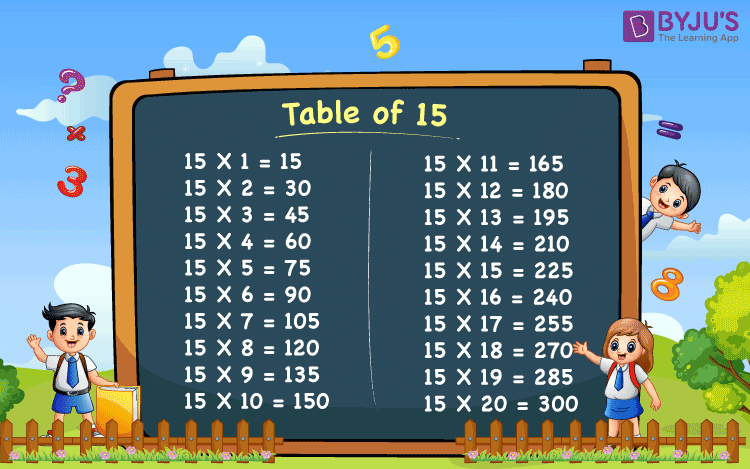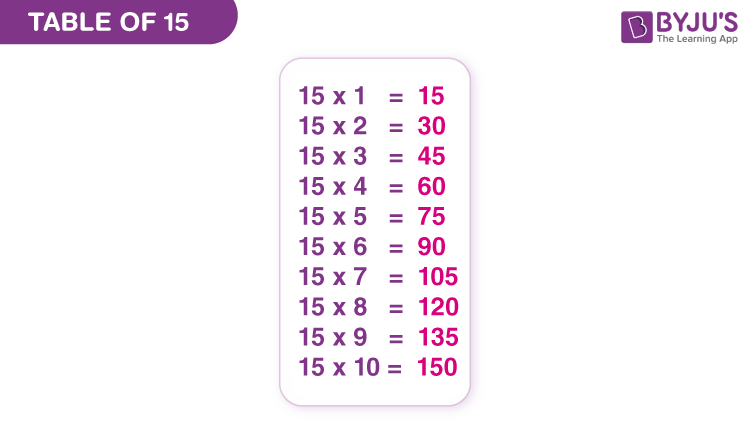# Table of 15

Table of 15 is the multiplication table of the whole number 15 that includes its multiples. 15 times table represents the repeated addition of number 15 to itself. For example, 15 multiplied by 3 is equal to 15 added to itself three times (15 x 3 = 15 + 15 + 15 = 45). It is necessary for students to memorise the table of 15 on finger-tips to solve complex multiplication problems. Tables from 2 to 15 are the base of the mathematical calculations, one must learn. Thus, it is required for students to remember these tables and increase their problem-solving capabilities.

## Table of 15 Chart

Multiplication table of 15 is one of the most common and easy multiplication tables. Among all the multiplication tables, the 15 times table can be easily memorized, which will help in the quick mathematical calculation.Table of 15 is provided in tabular form and in downloadable image format for the students.

## 2 Times Table up to 20 times

Learn the table of 15 up to 20 times here. This table will help students to solve complex multiplication problems quickly.

 15 × 1 = 15 15 × 2 = 30 15 × 3 = 45 15 × 4 = 60 15 × 5 = 75 15 × 6 = 90 15 × 7 = 105 15 × 8 = 120 15 × 9 = 135 15 × 10 = 150 15 × 11 = 165 15 × 12 = 180 15 × 13 = 195 15 × 14 = 210 15 × 15 = 225 15 × 16 = 240 15 × 17 = 255 15 × 18 = 270 15 × 19 = 285 15 × 20 = 300

From the above table, we can see, the table of 15 is very easy to learn. We have multiplied 15 by all the natural numbers from 1 to 20 to get its multiples. By downloading the 15 times multiplication table, students can learn the values more effectively and retain them for longer.

## Multiplication Table of 15## Tips to Memorise Table of 15

Although the 15 times table is easy to memorise, still some tips and tricks will help students to learn it quickly.

• The digits at the unit place are either 0 or 5
• The digits at tens place are such that, two consecutive odd numbers and then two consecutive even numbers. For example, the first two multiples 15 and 30 have odd numbers (i.e.,1 and 5) whereas the next two multiples 45 and 60 have even numbers (i.e.,4 and 6).
• Skip count for 15 to get the multiples of 15

## Solved Examples on Table of 15

Q.1: What is the value of 15 × 5 + 3?

Solution: Given,

15 × 5 + 3

= 75 + 3

= 78

Thus, the value of 15 × 5 + 3 is 78.

Q.2: Find: 15 × 4 – 15 × 2.

Solution: 15 × 4 – 15 × 2

= 60 – 30

= 30

Thus, 15 × 4 – 15 × 2 = 30.

Q.3: What is 15 times 3 minus 15 times 9?

Solution: From the table of 15, we have;

15 times 3 = 15 x 3 = 45

15 times 9 = 15 x 9 = 135

15 times 3 minus 15 times 9 = 45 – 135 = -95

## Practice Questions

Practice the worksheet given below, to check your knowledge on the table of 15.

1. 15 × 2 + 100 = ?
2. 15 × 8 – 60 = ?
3. 15 × 11 + 15 × 3 = ?
4. 15 × 15 = ?
5. 3 (15 × 10) = ?

## Frequently Asked Questions on Table of 15

Q1

### What is table of 15?

Table of 15 is the multiplication table that includes the multiples of 15. They are 15, 30, 45, 60, 75, 90, 105, 120, 135, 150.
Q2

### How to read the table of 15?

Table of 15 is read as:
15 ones are 15
15 twos are 30
15 threes are 45
15 fours are 60
and so on
Q3

### What is 15 times 6?

15 times 6 is equal to 90.
Q4

### What times of 15 is 180?

12 times 15 is 180.

Stay tuned with BYJU’S to get more Maths tables and learn other important Maths articles in a more effective and engaging way.

Test your knowledge on Table Of 15